Bihar Board 12th Maths Important Questions Long Answer Type Part 5

BSEB Bihar Board 12th Maths Important Questions Long Answer Type Part 5 are the best resource for students which helps in revision.

Bihar Board 12th Maths Important Questions Long Answer Type Part 5

Application Of Integrals

Question 1.
Find the area of the region in the first quadrant enclosed by the x-axis, the liney = x and the circle x2 + y2 = 32
Solution:
The given equations are
y = x …(i)
and x2 + y2 = 32 …(ii)
Solving (i) and (ii), we find that the line and’the-circle meet at B (4,4) in, the first quadrant., Draw perpendicular BM to the x- axis.
Therefore, the required area = area of the region OBMO + area of the region BMAB. ,
Now, the area of the region OBMO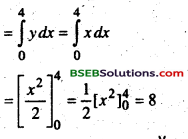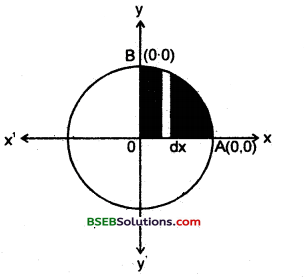Again, the area of the region BMAB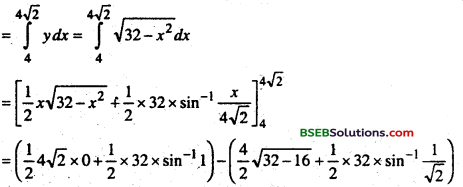Adding (iii) and (iv), we get
the required area = 8 + 4π – 8
= 4πQuestion 2.
Find the area bounded by the ellipse$$\frac{x^{2}}{a^{2}}+\frac{y^{2}}{b^{2}}$$ and the cordinates x = 0 and x = ae, where b2 = a2 (1 – e2) and e < 1.
Solution:
The required area of the region BOB’RFSB us enclosed by the ellipse and the line x = 0 and x = aeNow, the area of the region BOBRFSB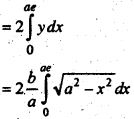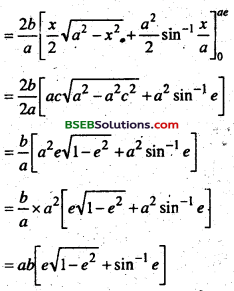Question 3.
Find the area of the parabola y2 = 4ax bounded by its locus rectum.
Solution:
The vertex of the parabola y2 = 4ax is at origin (0,0).
The equation of the locus rectum ∠SL’ is x = a. Also, parabola is symmetrical about the x-axis.
The required area of the region OLL’ = 2 (area of the region OLSO).Question 4.
Form the differential equation representing the family of curves y = a sin (x + b), where a, b are arbitrary constants.
Solution:
We have
y = a sin {x + b) ………….(i)
Differentiating both sides of the eqn. (i)
with respect to x, we get
$$\frac{d y}{d x}$$ = a cos (x + b) ……………… (ii)
$$\frac{d^{2} y}{d x^{2}}$$ = -a sin(x + b) ………………..(iii)
Eliminating a and b from equations
(i),(ii) and (iii), we get
$$\frac{d^{2} y}{d x^{2}}$$ + y = 0
Which is free form the arbitrary constants a and b hence this the required differential equation.

Question 5.
Find the general solution of the differential equation.
$$\frac{d y}{d x}=\frac{x+1}{2-y}$$, (y ≠ 2)
Solution:
We have
$$\frac{d y}{d x}=\frac{x+1}{2-y}$$
Separating the variables in equation (i), we get
(2-y)dy = (x + 1)dx
Integrating both sides of equation (ii), we get
∫(2-y)dy = ∫(x + 1)dx
2y – $$\frac{y^{2}}{2}=\frac{x^{2}}{2}$$+ x + c1
or, x2 + y2 + 2x – 4y + 2c = 0 .
or, x2 + y2 + 2x – 4y + c = 0,
Where c = 2c1
Which is the general solution of eqn. (i).

Question 6.
Find the particular solution of the differential equation
$$\frac{d y}{d x}=-4 x y^{2}$$ given that y = 1. where x = 0
Solution:
if y ≠ 0, the given differentail equation can be written as
$$\frac{d y}{y^{2}}$$ = -4x dx …………..(i)
Integrating both sides of equation (i), we get
$$\int \frac{d y}{y^{2}}$$ = -4∫x dx
$$-\frac{1}{y}$$ = -2x2 + c
y = $$\frac{1}{2 x^{2}-c}$$ ………….(ii)
Substituting y,= 1 and x = 0 in equation (ii), we get c = -1
Now substituting the value of c in equation (ii), we get the particular
solution of the given differential equation as y = $$\frac{1}{2 x^{2}+1}$$Question 7.
Show that the differential equation x – y $$\frac{d y}{d x}$$ = x + 2y is homogeneous and solve It.
Solution:
The given differential equation can be expressed as dy x + 2y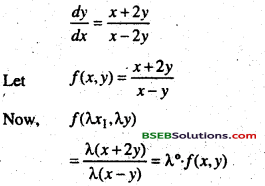Therefore, f(x, y) is a homogenous function of degree zero. So, the given differential equation is a homogenous differential equation.

Question 8.
Find the general solution of the differential equation
x$$\frac{d y}{d x}$$ + 2y = x2(x ≠ 0).
Solution:
The given differential equation is
x$$\frac{d y}{d x}$$ + 2y = x2
Dividing both sides of equation (i) by x, we get
$$\frac{d y}{d x}+\frac{2}{x} y=x$$
Which is a linear differential equation of the type
$$\frac{d y}{d x}$$ + py = Q
Where p = $$\frac{2}{x}$$ and Q = x
So, I.F $$\int_{e}^{2} \frac{2}{x} d x=e^{2 \log x}=e^{\log ^{x^{2}}}=x^{2}$$
Therefore, solution of the given equation is given by
y. x2 = ∫ (x)(x2) dx +c
= ∫x3 dx + c
= $$\frac{x^{2}}{4}$$ + cx-2
Which is the general solution of the given differential equation.

Question 9.
Solve the differential equation
(tan-1 y – x)dy = (1 + y2)dx
Solution:
The given differential eqn. can be written as
$$\frac{d x}{d y}+\frac{x}{1+y^{2}}=\frac{\tan ^{-1} y}{1+y^{2}}$$
Now (i) is a linear differential eqn. of die form $$\frac{d x}{d y}$$ + P1x = Q1
Where P1 = $$\frac{1}{1+y^{2}}$$ and Q1 = $$\frac{\tan ^{-1} y}{1+y^{2}}$$
Therefore, I.F = $$\int_{e} \frac{1}{1+y^{2} d y}=e^{\tan ^{-1} y}$$
Thus, the solution of the given differential eqn. is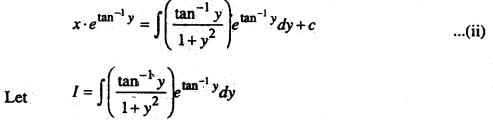substituting tan-1 y = t
So that ( $$\frac{1}{1+y^{2}}$$) dy = dt
I = ∫tetdt = tet – ∫1.etdt = tet – et
= et (t – 1)
I = etan-1(tan-1 y – 1)
Substituting the value of l in eqn. (ii), we get
x etan-1y = e tan-1 y(tan-1 y – 1) + c
or, x = (tan-1y – 1) + c e-tan-1y
Which is the general solution of the given differential equation.Question 10.
Find the area enclosed by the circle x2 + y2 = a2.
Solution:
The whole area enclosed by the given circle = 4 (area of the region AOBA bounded by the curve, x-axis and the ordinates x-0 and(x = a)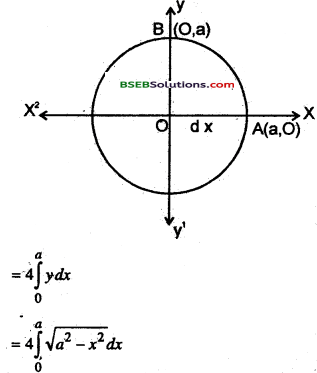As the region AOBA lies in the first quadrant y is taken as positive.
Integrating, we get the whole area enclosed by die given circle.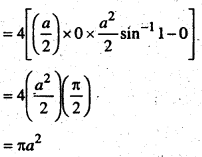Differential Equation

Question 1.
Solve x cos($$\frac{{y}}{{x}}$$)(ydx + xdy) = ysin($$\frac{{y}}{{x}}$$)(xdy – ydx).
Solution :
Put y = vx ⇒ dy = vdx + xdv
So the given equation becomes
x cos v(xvdx + xvdx + x2 dv) = vx sin v(yxdx + x2 dv – vxdx)
⇒ x2 cosv(2vdx+xdv) = vx2 sinvdv
⇒ 2vdx + xdv = vx tan vdv.
Dividing both sides by vac
⇒ $$\frac{2 d x}{x}+\frac{d v}{v}$$ = tan v dv. Intergrating, we get
2logx + logv = logseev + logc
x2v = cseev xy = c sec ($$\left(\frac{x}{y}\right)$$)

Question 2.
Solve (1 + y2)dx = (tan-1 y – x)dy.
Solution:$$\frac{d x}{d y}=\frac{\tan ^{-1}-x}{1+y^{2}} \Rightarrow \frac{d x}{d y}+\frac{x}{1+y^{2}}=\frac{\tan ^{-1} y}{1+y^{2}}$$ ……………. (1)
which is a linear equation of the form $$\frac{d x}{d y}$$ + px = Q.Multiplying (1) with I.F. arid integrating, we get ac
x x IF = ∫ Q x IF dyQuestion 3.
Solve (x + y)2$$\frac{d y}{d x}$$ = a2
Solution: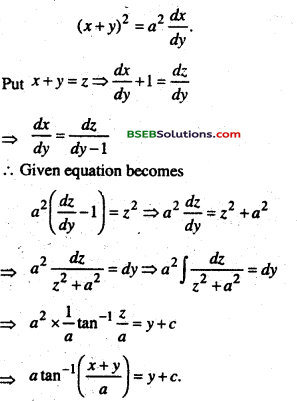Question 4.
Show that xdy – ydx = $$\sqrt{x^{2}+y^{2}} \cdot d x$$ is a homogeneous differential eqn. and hence solve it.
Solution:
Given eqn. xdy-ydx = $$\sqrt{x^{2}+y^{2}} \cdot d x$$ .dx
or, $$x \cdot \frac{d y}{d x}-y=\sqrt{x^{2}+y^{2}}$$……………..(i)
f(x) is homogeneous differential eqn
Put $$\frac{y}{n}$$ = v
y = vx
$$\frac{d y}{d x}=v+x \cdot \frac{d v}{d x}$$
From eqn (i)Question 5.
Solve -x $$\frac{d y}{d x}$$ = y. (log y – log x – 1)
Solution:
Given eqn is -x $$\frac{d y}{d x}$$ = y. (log y – log x – 1
or, $$\frac{d y}{d x}=\frac{y}{x} \cdot\left(\log \frac{y}{x}-1\right)$$
put v = $$\frac{y}{x}$$ ⇒ y = vx
∴ $$\frac{d y}{d x}=v+x \cdot \frac{d v}{d x}$$
From Eqn (i)
v + x $$\frac{d v}{d x}$$ = v. (log v -1)
or x.$$\frac{d v}{d x}$$ = v. (log v – 2)
or, $$\frac{d v}{v \cdot(\log v-2)}=\frac{d x}{\dot{x}}$$
or, $$\int \frac{d v}{v(\log v-2)}=\int \frac{d x}{x}$$
For L.H.S. put log v – 2 = t
1/v dv = dt
∴ From eqri. (ii),
$$\int \frac{d t}{f}=\int \frac{d x}{x}$$

or, log|t| = log|x| + log|c|
or, log|t| = log |x|; c
or, |t| = |x| .c
or, |log v — 2| = |x| c
or, |log($$\frac{y}{x}$$ – 2) = |x|.c
|log y – log x – 2| = c |x|Question 6.
Solve (x2 -1) $$\frac{d y}{d x}$$+2xy = $$\frac{2}{x^{2}-1}$$
Solution:Question 7.
Solve $$\frac{d y}{d x}$$ – 3y cot x = sin x given that y = 2 when x = $$\frac{\pi}{2}$$
Solution:
$$\frac{d y}{d x}$$ – 3y cot x = sin x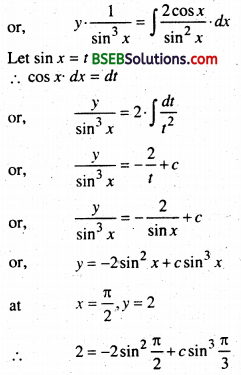or, 2 = -2 + c
c = 4
From eqn. (ii)
y = -2sin2x + 4sin3 xQuestion 8.
Solve $$\frac{d y}{d x}+\frac{1}{x}=\frac{e^{y}}{x}$$
Solution: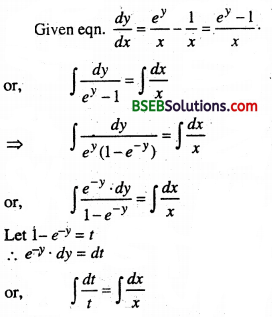⇒ iog|t| = log |x| + logc
⇒ log | 1 – e-y | = log |x| .c)
∴ 1 – e-y = c |x|

Question 9.
Solve (x + 2y3 )$$\frac{d y}{d x}$$ = y
Solution:
Given (x + 2y3 )$$\frac{d y}{d x}$$ = y
or, x + 2y3 = y$$\frac{d x}{d y}$$
or, y. $$\frac{d x}{d y}$$ – x = 2y3
or, $$\frac{d x}{d y}+\left(\frac{-1}{y}\right)$$. x = 2y2 …………. (i)
From eqn (i)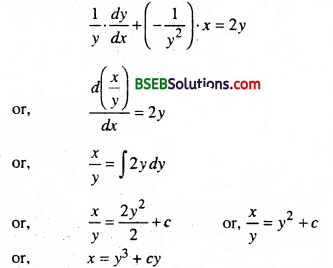Question 10.
$$\frac{d y}{d x}$$ + ycot x = 2y2 cos x
Solution:
$$\frac{1}{y^{2}} \frac{d y}{d x}+\frac{1}{y} \cot x$$ = 2cos x …………………..(1)
put $$\frac{1}{y}$$ ⇒ $$\frac{1}{y^{2}} \frac{d y}{d x}=\frac{-d z}{d x}$$
∴ (1) ⇒ $$\frac{-d z}{d x}$$ + zcotx = 2cos x
$$\frac{d z}{d x}$$ z cot x = -2 cos x
∴ I.F = e-∫cotxdx = e-logsinx = cosec x
Multiplying (2) by IF and integrating, we have
zx cosec x = -∫ cosx . cosec xdx = -2 ∫ cot x dx
⇒ z cosec x = -2 log sin x + k ⇒ $$\frac{cosecx}{y}$$ = -2logsin x + kQuestion 11.
$$\frac{d y}{d x}$$ + y sec x = tan x
Solution:
I. F = e∫secx dx = e∫log(sec+tanx) = sec x + tan x
Multiplying the given eqn. with I.F.and integrating we get.
∴ y x (sec x + tan x) =∫ tanx(secx + tanx)dx
= ∫ tanx secx dx + ∫ (sec2x – 1)dx
⇒ y(secx + tan x) = sec x + tan x – x + k.

Question 12.
Evaluate ∫sec3 xdx
Solution:
I = ∫sec3 xdx = ∫sec2 x.sec xdx
⇒ I = secx∫sec2xdx – ∫ [$$\frac{d}{d x}$$ (secx)∫sec2xdx]dx
= secx tanx – ∫secx – tanx tanxdx
= sec x tanx- ∫sec x(sec2 x – 1)dx
= sec x tan x – ∫sec3 xdx + ∫secdx
= secx tanx – I + log(secx + tanx) + k
⇒ 2I = sec x . tan x + log (secx + tan x) + k
⇒ I = $$\frac { 1 }{ 2 }$$ secx tanx + $$\frac { 1 }{ 2 }$$log(secx + tanx) + c .

Question 13.
Evaluate ∫cos$$\sqrt{x} d x$$
Solution:
Let $$\sqrt{x} d x$$ = z
⇒ x = z2 ⇒ dx = 2zdz
∴ I = ∫cos z. 2zdz = 2 ∫zcoszdz
= 2z∫coszdz – 2∫$$\frac{d}{d z}$$(z)∫ coszdz]dz
= 2zsinz- 2∫sinzdz
= 2 z sinz + 2 cos z + c
⇒ I = $$2 \sqrt{x} \sin \sqrt{x}+2 \cos \sqrt{x}$$ + c

For each of the differential equations in exercises from 14 to 17, find a particular solution satisfying the given condition.

Question 14.
(x3 + x2 + x + x + 1)$$\frac{d y}{d x}$$ = 2x2 + x; y = 1 when x = 0
Solution:
(x3 + x2 + x + x + 1)$$\frac{d y}{d x}$$ = 2x2 + x;
or, (x3 + x2 + x + 1)dy = (2x2 + x )dx2x2 + x = A(x2 + 1) + (Bx + C)(x + 1)
= A(x2 +1) + B(x2 + x) + C( x + 1)
Put x = -1, 2 – 1 = A( 1 + 1) ⇒ A = $$\frac{1}{2}$$
Comparing the cofficients of x2 and x
2 = A + B and 1 = B + C
B = 2 – A = 2 – $$\frac{1}{2}=\frac{3}{2}$$
C = 1, B = 1 – $$\frac{3}{2}=-\frac{1}{2}$$[Note : for integrating $$\int \frac{2 x}{x^{2}+1} d x$$, put x2 + 1 = t 1
We have y = 1 when x = 0
Putting these values
1 = $$\frac{1}{2}$$logl + $$\frac{3}{4}$$log1 – $$\frac{1}{2}$$ tan-10 + c
= 0 + c is c = 1
Thus the solution is
y = $$\frac{1}{2}$$ log(x + 1) + $$\frac{3}{4}$$ (x2 + 1) – $$\frac{1}{2}$$ tan-1 x + 1
= $$\frac{1}{4}2$$2log(x + 1) + $$\frac{3}{4}$$log(x2 + 1) – $$\frac{1}{2}$$ tan-1 x + z
= $$\frac{1}{4}$$[Iog(x + 1)2 + log(x2 + 1)3] – tan-1 x + 1
= $$\frac{1}{4}$$ log(x + 1)2(x2+1)2 – $$\frac{1}{2}$$tan-1 x + 1
This is the required solution.Question 15.
x(x2 – 1)dy/dx = 1
Solution:
x(x2 – 1)dy/dx = 1
or x(x2 – 1)dy = dx
∴ $$d y=\frac{d x}{x\left(x^{2}-1\right)}=\frac{d x}{x(x-1)(x+1)}$$
Integrating, we get1= A (x – 1) (x + 1) + Bx (x + I) + Cx (x-1)
Put x-0,1 = A (-1)(+1) ∴ A = -1
Put x = 1, 1 = B = 1.2 ∴ B = $$\frac{1}{2}$$
Put x = -1, 1 = C (-1) (-2) ∴ C = $$\frac{1}{2}$$
∴ y = $$\int \frac{d x}{x}+\frac{1}{2} \int \frac{1}{x-1} d x+\frac{1}{2} \int \frac{d x}{x+1}$$
= – log x + $$\frac{1}{2}$$ log; (x -1) + $$\frac{1}{2}$$ log(x +1) + log C
Multing by 2
2y = -2 log x + log (x – 1) + log (x + 1) + 2 log C.
= log $$\frac{x^{2}-1}{x^{2}}$$ + 2 log C.
When x = 2, y = 0
∴ 0 = log $$\frac{4-1}{4}$$ + 2 log C.
2 log C = -log$$\frac{3}{4}$$
Solution isQuestion 16.
cos $$\frac{d y}{d x}$$ = a; (a ∈ R), y = 1 when x = 0
Solution:
cos $$\frac{d y}{d x}$$ = a ∴ $$\frac{d y}{d x}$$ = cos-1a
or dy = (cos-1a)dx
Integrating
∫ dy = (cos-1a)∫dx or, y = (cos-1a)x + C
we have y = 2 when x = 0
2 = C
∴ Solution y = x(cos-1a) + 2 or cos-1 a = $$\frac{y-2}{x}$$
a = cos $$\frac{y-2}{x}$$ is the reqd. solution.

Question 17.
Solution:
$$\frac{d y}{d x}$$ = ytan x; y = 1 when x = 0
Solution:
$$\frac{d y}{d x}$$ = ytan x
or, $$\frac{d y}{y}$$ = tan xdx
Integrating we get
$$\int \frac{d y}{y}=\int \tan x d x$$
log y = – log cos x + log C
or, log y + log cos x = log C
or, lyg y cosx = log C
∴ y cos x = C
Putting y = 1, x = 0 => C = 1
∴ y cos x = 1
or, y = sec x is the solution.